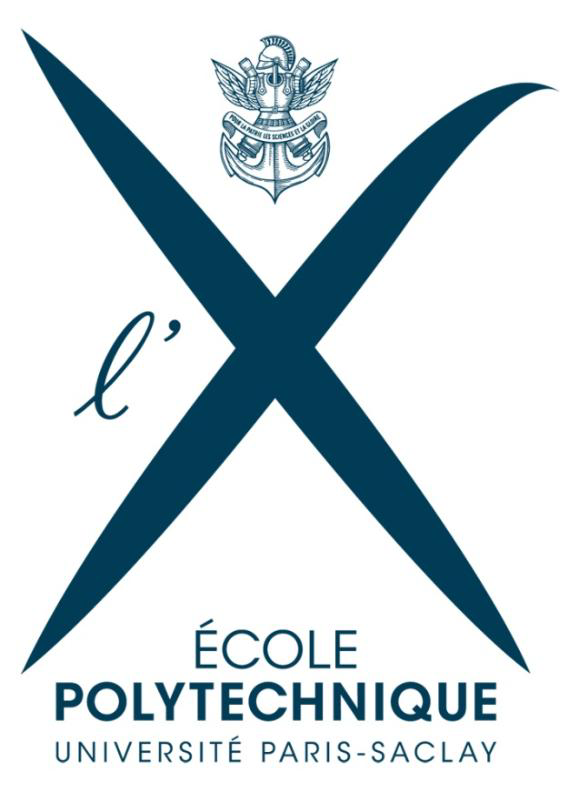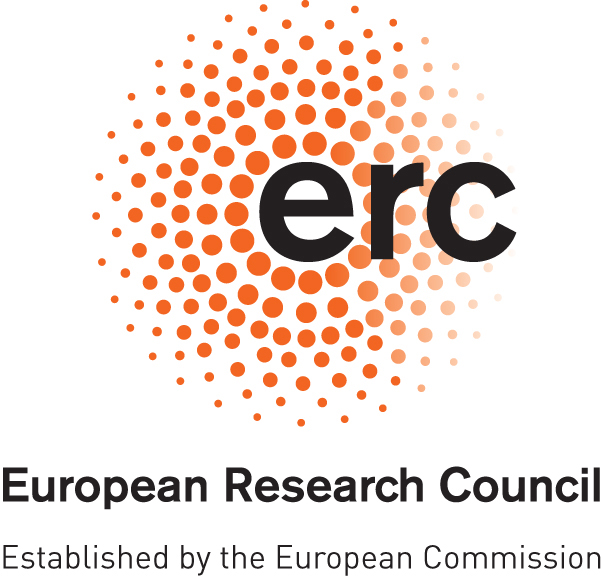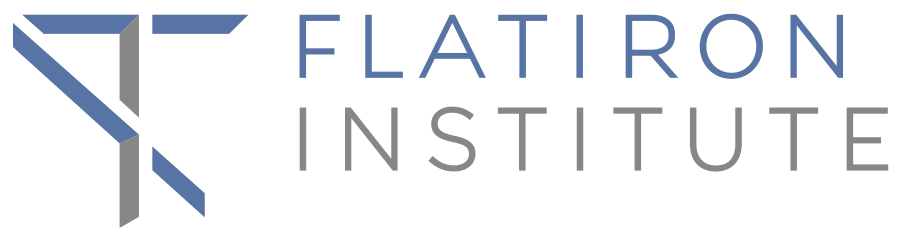# The hybridization-expansion solver

The TRIQS-based hybridization-expansion solver allows to solve the generic problem of a quantum impurity embedded in a conduction bath for an arbitrary local interaction vertex. The “impurity” can be any set of orbitals, on one or several atoms. To be more specific, the Hamiltonian of the problem has the form

$\hat H = \sum_{k,\alpha} \epsilon_{k,\alpha} c^\dagger_{k,\alpha} c_{k,\alpha} + \sum_{k,\alpha} (V_{k,\alpha} c^\dagger_{k,\alpha} d_{\alpha} + h.c.) - \mu \sum_\alpha d^\dagger_\alpha d_\alpha + \sum_{\alpha\beta} h_{\alpha\beta} d^\dagger_\alpha d_\beta + \frac{1}{2}\sum_{\alpha\beta\gamma\delta} U_{\alpha\beta\gamma\delta} d^\dagger_\alpha d^\dagger_\beta d_\delta d_\gamma.$

Here the operators $$c^\dagger$$ construct a fermion in the bath, while the operators $$d^\dagger$$ construct a fermion on the impurity. In this problem, the hybridization function $$\Delta$$ between the bath and the impurity is given by:

$\Delta_{\alpha\beta} (i \omega_n) = \sum_k \frac{V_{k,\alpha} V^*_{k,\beta}}{i \omega_n - \epsilon_{k,\alpha}},$

so that the non-interacting Green’s function of the impurity is:

$\hat{G}^{-1}_0 (i \omega_n) = i \omega_n + \mu - \hat h - \hat{\Delta}(i \omega_n).$

With the knowledge of $$G_0$$ and the matrix $$U_{\alpha\beta\gamma\delta}$$, the quantum impurity solvers find the interacting Green’s function $$G$$ of the problem. Learn how to use it in the Documentation.